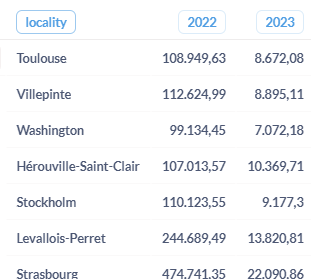# Using field referenced by a variable

Hello,

I’m using Metabase (dashboarding tool), that allows to write queries in MongoDB language. I have a collection containing a locality, a year (in number format) and an amount. I wrote a query that accepts an input variable for the year. Based on that input variable, the query first applies a filter and selects the documents matching the year provided or the previous year (so if I provide 2023 in input, it selects the documents for which the year is equal to 2023 or 2022). Then I do a sum of amounts grouped by locality and year, and I apply some pivot transformations so that the years become new fields. At the end I have something like this :I would like to add a new column containing the difference between the sum of 2023 and the sum of 2022, for each locality. If I simply do this, it works fine:

``````{
diff: { \$subtract: [ "\$2023", "\$2022"] }
}
},
``````

However, I cannot hardcode the field names like I did, since they depend on the value that I provided in the filter at the beginning. Basically, the field “2023” corresponds to {{yearFilter}} (the double brackets is how the filters are represented in Metabase), and the field “2022” corresponds to {\$subtract: [ {{yearFilter}}, 1] }.
So I tried to use those patterns in the code above:

``````    {
"diff": {
\$subtract: [
"\${{yearFilter}}",
"\${\$toString: {\$subtract: [ {{yearFilter}}, 1] } }"
]
}
}
},
``````

But it doesn’t work. I see the new column, but it is empty. In order to understand where the problem comes from, I did the following:

``````{
YEAR1test: "\${{yearFilter}}"
}
},
{
"YEAR2test": {\$toString: {\$subtract: [ {{yearFilter}}, 1] } }
}
},
{